## Example Questions

### Example Question #4 : Variables

Multiply: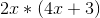Possible Answers: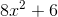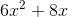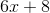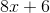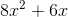Correct answer:Explanation:

To solve you must multiplyby both terms in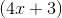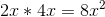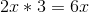### Example Question #5 : Variables

Multiply: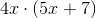Possible Answers: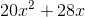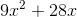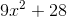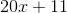Correct answer:Explanation:

Multiply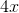by both terms in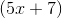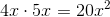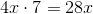### Example Question #6 : Variables

Multiply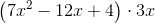Possible Answers: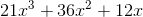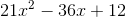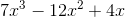None of the other answers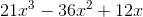Correct answer:Explanation:

When multiplying a polynomial by a monomial, each term in the polynomial gets multiplied by the monomial. Calculate each term one at a time, then add the results to get the final answer. In this case, we start by multiplying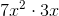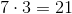and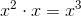, thus we get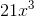. For the second term of the polynomial, we multiply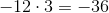and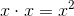, resulting in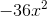. Finally, we multiply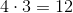and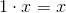, resulting in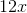. Adding the three terms that we just found, we come to the answer of.

### All ACT Math Resources